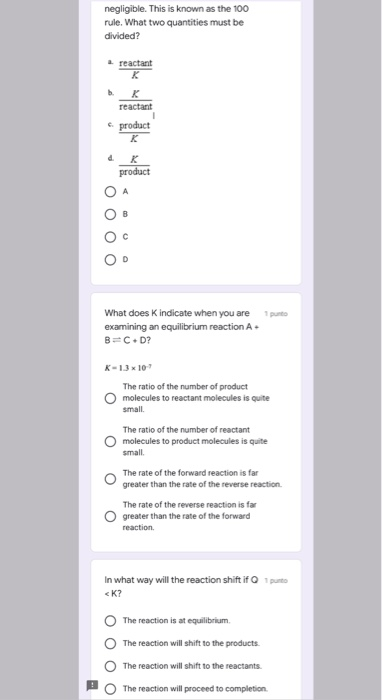# Question Solved1 Answernegligible. This is known as the 100 rule. What two quantities must be divided? reactant b. reactant e product K d. K product puto What does K indicate when you are examining an equilibrium reaction A. B=C+D? K-13x10 The ratio of the number of product molecules to reactant molecules is quite small The ratio of the number of reactant molecules to product molecules is quite small The rate of the forward reaction is far greater than the rate of the reverse reaction The rate of the reverse reaction is far greater than the rate of the forward reaction In what way will the reaction shift if punto <K? The reaction is at equilibrium The reaction will shift to the products 0 The reaction will shift to the reactants O The reaction will proceed to completionTranscribed Image Text: negligible. This is known as the 100 rule. What two quantities must be divided? reactant b. reactant e product K d. K product puto What does K indicate when you are examining an equilibrium reaction A. B=C+D? K-13x10 The ratio of the number of product molecules to reactant molecules is quite small The ratio of the number of reactant molecules to product molecules is quite small The rate of the forward reaction is far greater than the rate of the reverse reaction The rate of the reverse reaction is far greater than the rate of the forward reaction In what way will the reaction shift if punto
More
Transcribed Image Text: negligible. This is known as the 100 rule. What two quantities must be divided? reactant b. reactant e product K d. K product puto What does K indicate when you are examining an equilibrium reaction A. B=C+D? K-13x10 The ratio of the number of product molecules to reactant molecules is quite small The ratio of the number of reactant molecules to product molecules is quite small The rate of the forward reaction is far greater than the rate of the reverse reaction The rate of the reverse reaction is far greater than the rate of the forward reaction In what way will the reaction shift if punto